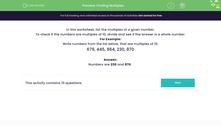# Identify Multiples

In this worksheet, students will work through a number problem to find the multiples.Key stage:  KS 2

Curriculum topic:   Number: Multiplication and Division

Curriculum subtopic:   Solve Multiplication/Division Problems

Difficulty level:#### Worksheet Overview

We will be looking at the multiples of numbers in this activity.

Here are the first five multiples of six: 6, 12, 18, 24, and 30

Here are the first five multiples of nine: 9, 18, 27, 36 and 45

The list of multiples of a number don't stop just because we reach our twelve times table!! They go on forever!There are sometimes easy ways to spot if a number is a multiple of another number.

For example:

Any multiple of 10 will end in a zero.

Multiples of 5 end in a zero or a 5.

Multiples of 2 end in an even number: 0, 2, 4, 6 or 8

In the list of numbers below, which ones are multiples of 2, 5 or 10?

679, 445, 964, 230, 870

Multiples of 2: these must end in an even number, so that is 964, 230 and 870.

Multiples of 5: these end in a zero or a 5, so that is 445, 230 and 870.

Multiples of 10: these end in a zero, so 230 and 870

Was that simple?In the following questions, there will also be some harder ones to solve where we will have to do a division calculation. If there is a remainder in our calculation then the number we've divided can't be a multiple of our given number.

Let's give it a go!

### What is EdPlace?

We're your National Curriculum aligned online education content provider helping each child succeed in English, maths and science from year 1 to GCSE. With an EdPlace account you’ll be able to track and measure progress, helping each child achieve their best. We build confidence and attainment by personalising each child’s learning at a level that suits them.

Get started